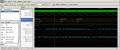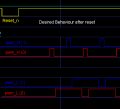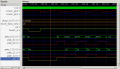# Complimentary outputs going hi after reset - VHDL

#### tonigau

Joined May 2, 2011
18
Still learning VHDL... [ using GHDL V2.0, gtkwave V3.3 & UMHDL IDE V2.50b]

I have modified a VHDL example to include dead-time between complimentary outputs & it works well, except for a couple of issues.
1. The pwm_L outputs go hi after 'Reset_n' 0>1 (active lo reset)
2. After 'Reset_n' 0->1 'pwm_L(0)' output is active before pwm_h

I have done a lot of re-arranging, modifying & tests but can't clik_on why 'pwm_L' output is behaving different to 'pwm_H'.
If I swap '_L' & '_H' in the snippet code the issue(s) follow... [after a few weeks I might find it]

Desired state after reset (see smaller attached image)
All 'pwm_x(x)' outputs at 0 untill count condition is true (as it is with pwm_H )
The pwm_H hi pulse must precede pwm_L hi pulse, which is observed after the second reset in the attached simulation.
After the second reset release (175us) the order of phases is also correct - ph1 before ph2 .

--------------------------------
. Notes: The testbench is hard coded for some generic values in UUT.
To load the .gtkw simulation file, first edit the 'dumpfile' & 'savefile' path in the .gtkw & drag-drop onto gtkw, this will setup gtkw wave formats, then clik refresh button when build/sim from ghdl .

snippet - PWM outputs:
FOR i IN 0 to phases-1 LOOP
IF(Count(i) = Half_Duty(i)) THEN
pwm_H(i) <= '0';
ELSIF(Count(i) = period - Half_Duty(i)) THEN
pwm_H(i) <= '1';
END IF;
--Insert DT between complementary out.
IF(Count(i) = Half_Duty(i) + DT_cnt) THEN
pwm_L(i) <= '1';
ELSIF(Count(i) = period - DT_cnt - Half_Duty(i)) THEN
pwm_L(i) <= '0';
END IF;
END LOOP;#### Attachments

• 585 bytes Views: 0
• 5.6 KB Views: 1
• 2.6 KB Views: 1
• 119 KB Views: 0
Last edited:

#### tonigau

Joined May 2, 2011
18
ok, for a test I swapped the states '0' '1' in the if condition for PWM_L(x) [snippet code --Set PWM outputs].
Code:
IF(Count(i) = Half_Duty(i) + DT_cnt) THEN
pwm_L(i) <= '0';
ELSIF(Count(i) = period - DT_cnt - Half_Duty(i)) THEN
pwm_L(i) <= '1';
END IF;
& PWM_L outputs behave more as expected (except being inverted of course).
For the condition of PWM_L(x) becoming true before PWM_H(x) after reset seems to be due to count(x) condition being a match to Half_Duty(x).
but why both PWM_L(x) became true after Reset_n may be the due to 'IF condition state' already existed? before reset release.

So I need to restructure the PWM_L(x) out condition code.

Any help to improve my understanding & approach to achieve result would be welcome.
My 35+ years of uC programming is not helping me a lot with VHDL behavior.

-------------------------------------------
If I change the code to the following, the PWM_L output is just like the code snippet in first post (without DeadTime of course) & doesn't just create inverse PWM_L(x) outputs.

Test...:
     FOR i IN 0 to phases-1 LOOP
IF(Count(i) = Half_Duty(i)) THEN
pwm_H(i) <= '0';
pwm_L(i) <= '1';
ELSIF(Count(i) = period - Half_Duty(i)) THEN
pwm_H(i) <= '1';
pwm_L(i) <= '0';
END IF;

#### tonigau

Joined May 2, 2011
18
I devised a way to disable pwm_L(x) outputs after reset...
Create a signal 'EN_L'
set EN_L <= False; on reset
set EN_L <= True; inside pwm_H code block
Test for EN_L=True before set pwm_L(x)

At first I tried to test the port state but Elaboration couldn't resolve a not yet defined state.

---> Still need to find out why the first reset affects order of phases !!!
Edit: after some more testing... its the 'SIGNAL Half_Duty' starting at value 0

-------------------------
It's not unlike fixing a fault on a circuit board, can spend a lot of time finding & it can be just a component that has drifted out of circuit tolerance -- minutes to fix.Last edited:

#### drjohsmith

Joined Dec 13, 2021
549
You have done well there,
But, I am guessing your a C programmer,

The for loop, is not working as you are thinking,
Try looking at the generate statement,

Also ,
never use the synopsis library ,
it will only give you grief ,

Where are you learning VHDL from

#### tonigau

Joined May 2, 2011
18
I understand the for loops are saving me to enter repeating code lines...

Expanded for loop:
   IF(Count(0) = Half_Duty(0)) THEN pwm_H(0) <= '0';
ELSIF(Count(0) = period - Half_Duty(0)) THEN pwm_H(0) <= '1'; EN_L <= True;
END IF;

IF(Count(1) = Half_Duty(1)) THEN pwm_H(1) <= '0';
ELSIF(Count(1) = period - Half_Duty(1)) THEN pwm_H(1) <= '1'; EN_L <= True;
END IF;
>> "never use the synopsis library , it will only give you grief ,"

I had to include the synopsis just to get it working so its a "just for now", it gets worse...
I had this code: (2**n_bits) to get the max value from bits assigned. All good
I needed to do the inverse LOG(Duty)/LOG(2) & the fun started, had to convolute into
natural := integer(ceil(log2(real(phases))))

So I will just hard code the number of bits

I learn mostly by just coding, when I get stuck I ask the white duck.

#### drjohsmith

Joined Dec 13, 2021
549
I understand the for loops are saving me to enter repeating code lines...

Expanded for loop:
   IF(Count(0) = Half_Duty(0)) THEN pwm_H(0) <= '0';
ELSIF(Count(0) = period - Half_Duty(0)) THEN pwm_H(0) <= '1'; EN_L <= True;
END IF;

IF(Count(1) = Half_Duty(1)) THEN pwm_H(1) <= '0';
ELSIF(Count(1) = period - Half_Duty(1)) THEN pwm_H(1) <= '1'; EN_L <= True;
END IF;
>> "never use the synopsis library , it will only give you grief ,"

I had to include the synopsis just to get it working so its a "just for now", it gets worse...
I had this code: (2**n_bits) to get the max value from bits assigned. All good
I needed to do the inverse LOG(Duty)/LOG(2) & the fun started, had to convolute into
natural := integer(ceil(log2(real(phases))))

So I will just hard code the number of bits

I learn mostly by just coding, when I get stuck I ask the white duck.
Remember,